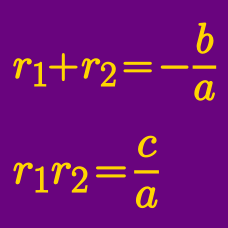Algebra

# Vieta's Formula: Level 5 Challenges

If the roots of $$p(x) = x^3 + 3x^2 + 4x - 8$$ are $$\color{red}{a}$$, $$\color{blue}{b}$$ and $$\color{purple}{c}$$, what is the value of

$\color{red}{a}^2 \big(1 + \color{red}{a}^2\big) + \color{blue}{b}^2 \big(1 + \color{blue}{b}^2\big) + \color{purple}{c}^2 \big(1 + \color{purple}{c}^2\big)?$

If $$\alpha , \beta , \gamma$$ are roots of the equation $$x^{3} + 3x + 9 = 0,$$ find the value of $$\alpha^{9} + \beta^9 + \gamma^{9}.$$

Consider all pairs of non-zero integers $$(a,b)$$ such that the equation

$( ax-b)^2 + (bx-a)^2 = x$

has at least one integer solution.

The sum of all (distinct) values of $$x$$ which satisfy the above condition can be written as $$\frac{ m}{n}$$, where $$m$$ and $$n$$ are coprime positive integers. What is the value of $$m + n$$?

Let $$x_1,x_2,\ldots,x_{2015}$$ be the roots of the equation $x^{2015} + x^{2014} + x^{2013} + \ldots + x^2 + x + 1 =0.$ Evaluate

$\frac1{1-x_1} + \frac1{1-x_2} + \ldots + \frac1{1-x_{2015}}.$

What is the largest integer $$n \leq 1000$$, such that there exist 2 non-negative integers $$(a, b)$$ satisfying

$n = \frac{ a^2 + b^2 } { ab - 1 } ?$

Hint: $$(a,b) = (0,0)$$ gives us $$\frac{ 0^2 + 0^2 } { 0 \times 0 - 1 } = 0$$, so the answer is at least $$0 .$$

×

Problem Loading...

Note Loading...

Set Loading...